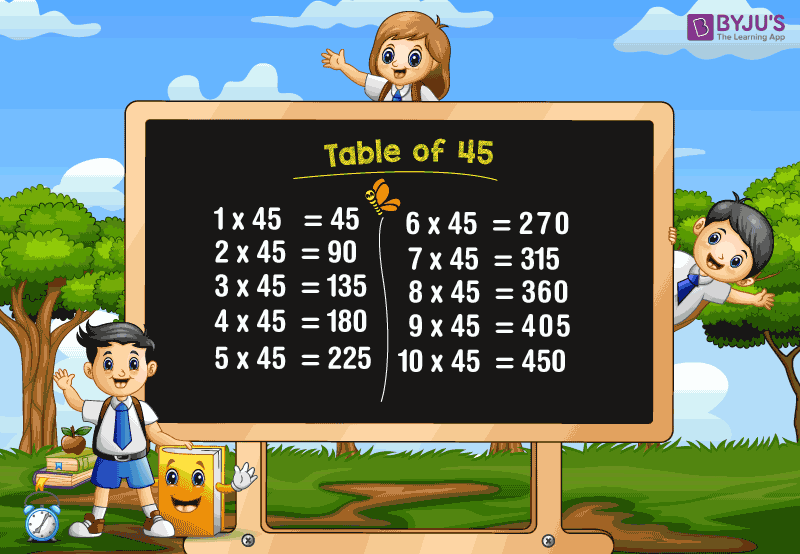# Table of 45

Table of 45 is a Maths multiplication table for the number forty-five (45) and memorising this table helps in solving numerical problems based on multiplication, division and square roots. Students who are preparing for competitive exams are advised to learn these Tables from 1 to 100 so that they can save the time of simplifications.

### Download Table of 45 HereWhat is the table of 45?

The table of 45 can be defined using the repeated addition of 45. For example, a group of students sat around 45 tables so that each table was surrounded by 4 students. In this case, the total number of students can be calculated using the multiplication table.

That means, each of the 45 tables surrounded by 4 students.

Thus, the total number of students = 45 × 4 = 180

This can also be expressed as, 45 + 45 + 45 + 45 = 180

Hence, we can get all the multiples of 45 as explained above. However, the table of multiplication of forty-five from 1 to 20 is given below.

## Multiplication Table of 45

 45 × 1 = 45 45 × 2 = 90 45 × 3 = 135 45 × 4 = 180 45 × 5 = 225 45 × 6 = 270 45 × 7 = 315 45 × 8 = 360 45 × 9 = 405 45 × 10 = 450 45 × 11 = 495 45 × 12 = 540 45 × 13 = 585 45 × 14 = 630 45 × 15 = 675 45 × 16 = 720 45 × 17 = 765 45 × 18 = 810 45 × 19 = 855 45 × 20 = 900

### How to Read Table of 45

Read the forty-five times table from 1 to 10 as given below.

• One time forty-five is forty five
• Two times forty-five is ninety
• Three times forty-five is one hundred and thirty five
• Four times forty-five is one hundred and eighty
• Five times forty-five is two hundred and twenty five
• Six times forty-five is two hundred and seventy
• Seven times forty-five is three hundred and fifteen
• Eight times forty-five is three hundred and sixty
• Nine times forty-five is four hundred and five
• Ten times forty-five is four hundred and fifty

### Get More Tables

To learn more multiplication tables, visit www.byjus.com and download BYJU’S – The Learning App for interactive and engaging videos.